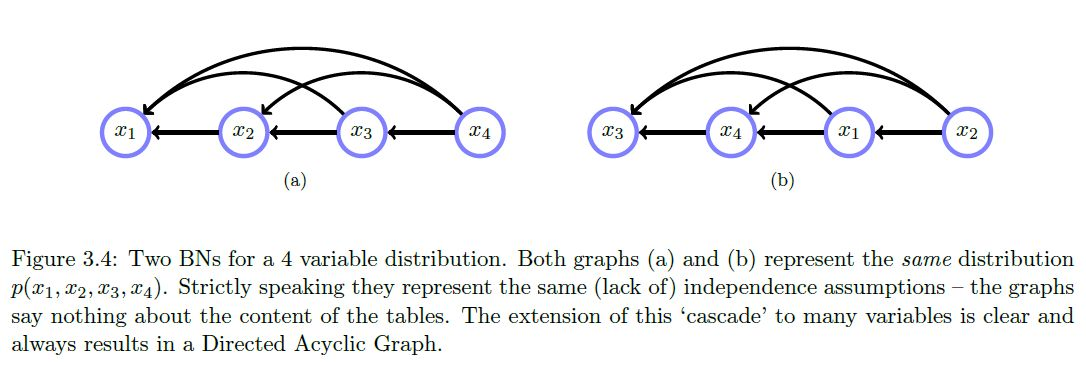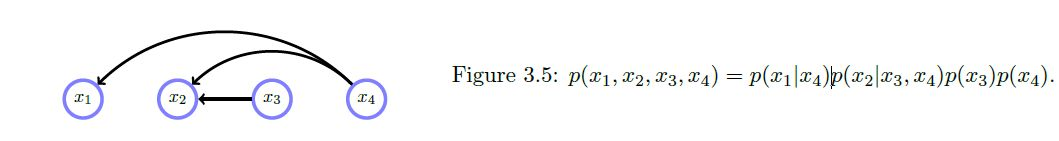Show Question
Math and science::INF ML AI

# Belief networks

A belief network (called a Bayesian network by Koller) is a grapical model that represents a distribution of the form
$p(x_1, ..., x_D) = \prod_{i=1}^{D} p(x_i \vert pa(x_i)) \\\text{where } pa(x_i) \text{ represents the parental variables of variable } x_i$

Parental variables is just a term for the variables that appear as conditional variables for a given variable.

A belief network represents a distribution as a directed graph where the $$i$$th node corresponds to the factor $$p(x_i \vert pa(x_i))$$, with directed connections from all $$pa(x_i)$$ nodes to the $$i$$th node.

According to the rules above, a distribution $$p(x_1, x_2, x_3, x_4)$$, one with no independence statements, can be modelled with a cascade graph like so:### Derivation

Without loss of generality, using Bayes' rule the distribution $$p(x_1, ..., x_n)$$ can be represented as:

\begin{aligned} p(x_1, ..., x_n) &= p(x_1 \vert x_2, ..., x_n)p(x_2, ..., x_n) \\ &= p(x_1 \vert x_2, ..., x_n)p(x_2 \vert x_3, ..., x_n)p(x_3, ..., x_n) \\ &= p(x_n) \prod_{i=1}^{n-1} p(x_i \vert x_{i+1}, ..., x_n) \end{aligned}

More accurately, a belief network represents a set of distributions that have the same dependency relationships, not just a single distribution with speficied probabilities.

### Example

Deleting any connection represents the existance of some independence. For example:#### Source

Bayesian Reasoning and Machine Learning
David Barber
p38-39